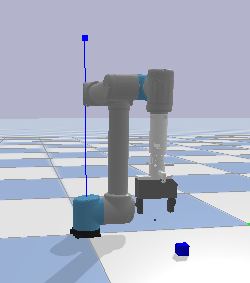# Improve performance of my model for planning robot re-grasping

Hi,

my name is Robin and I’m completely new to PyTorch. I’m working in university project where we a robot arm is grasping an object. Our goal is to check, if the data measured while grasping is enough to detect, whether the grab is good or should be replanned.

Therefore, I’ve implemented the robot and his movement actions in PyBullet (physics engine). First, the robot is moving to the object and closing its fingers. At this point, I measure the 3 forces and 3 moments. Then a re-grab is performed with little angular deviation for all 3 axis for a better (expected) grip. The object to be grabbed is a simple cube, which position and orientation is completely random, but within the reach of the robot arm.My problem:
I’ve trained a few models with different parameters, for example to get best-performing learning rate. My latest version of the model (around 250 steps with batch size of 25 each) gave a relatively good loss over time.

But when I try to evaluate with graphical rendering, it seem’s like my approach is not working (so far), the suggested angular deviations for re-grabbing are not really good.

My question is: What are the steps to get a better performance? What do I have to check/ maybe debug? Which parameters might need to be changed a bit? Is my way of calculating the reward good or should I follow another approach? I think here is enough experience to give me some tippsThe model class is implemented this way:

``````class QLearningNN(nn.Module):

def __init__(self):
super().__init__()

self.input_1 = nn.Linear(6,20)
self.input_2 = nn.Linear(3,10)

self.hidden_1 = nn.Linear(30, 30)
self.hidden_2 = nn.Linear(30,20)
self.hidden_3 = nn.Linear(20,10)

self.output_layer = nn.Linear(10,1)

def forward(self, x1, x2):
x1 = torch.tanh(self.input_1(x1))
x2 = torch.tanh(self.input_2(x2))
x = torch.cat((x1, x2), dim=1)

x = self.hidden_1(x)
x = self.hidden_2(x)
x = self.hidden_3(x)

x = self.output_layer(x)

return x
``````

So, my inputs are the 6-dim vector of the 3 forces/ moments of the prior grip and also a 3-dim vector with little angular deviations. Output is the expected reward. I want to perform q-Learning.

The ‘actions’ (= angular deviation for 3 axes) are defined as:

``````def get_all_actions(self):
return  [(dx*pi/180, dy*pi/180, dz*pi/180) for dx in range(-10,10,2) for dy in range(-10,10,2) for dz in range(-10,10,2)]
``````

reward is calculated this way:

``````def calculate_reward(self, gripperData):
cube, _ = self.objects
cubeQuat = p.getBasePositionAndOrientation(cube)
cubeMat = p.getMatrixFromQuaternion(cubeQuat)
cubeMat = np.array([cubeMat[:3], cubeMat[3:6], cubeMat[6:9]])

# GuideMat * M = CubeMat
# <=> M = GuideMat^-1 * CubeMat

guideQuat = gripperData.eeGuideOrnQuat.get_as_list()
guideMat = p.getMatrixFromQuaternion(guideQuat)
guideMat = np.array([guideMat[:3], guideMat[3:6], guideMat[6:9]])

guide_inv = np.linalg.inv(guideMat)
diff_mat = np.matmul(guide_inv, cubeMat)

thetaX = np.arctan2(diff_mat[2,1], diff_mat[2,2])
thetaY = np.arctan2(-diff_mat[2,0], np.sqrt(diff_mat[2,1]**2 + diff_mat[2,2]**2))
thetaZ = np.arctan2(diff_mat[1,0], diff_mat[0,0])

return -1*np.sqrt(thetaX ** 2 + thetaY ** 2 + thetaZ ** 2)
``````

The object to be grabbed is the cube. I get the cube orientation matrix and calculated the needed matrix M, so that GuideMat * M = CubeMat (Where GuideMat is a coordinate system between the fingers of the robot). Reward is negative so that I can max it.

Last bit of code is my model_optim method:

``````def optimize_model(self):
if len(self.memory.data) < self.batch_size: return

state, action, target_reward = self.memory.get_samples()

self.model.train()

reward = self.model(state, action)

criterion = nn.SmoothL1Loss()
loss = criterion(reward, target_reward)
self.log_loss(loss)

loss.backward()
self.optimizer.step()
torch.save(self.model, self.model_name)
``````

I hope everything needed to understand the problem is there - otherwise I’ll explain more detailed.

Thanks a lot!

P.S.: After reading through my lines I feel a bit dump; My possible actions are within 10 degrees angular deviation but the object could be placed randomly…

P.P.S.: What is the definition of this model? Q-Learning with a regression model with discrete and continuous (actions discrete, forces continuous) inputs?